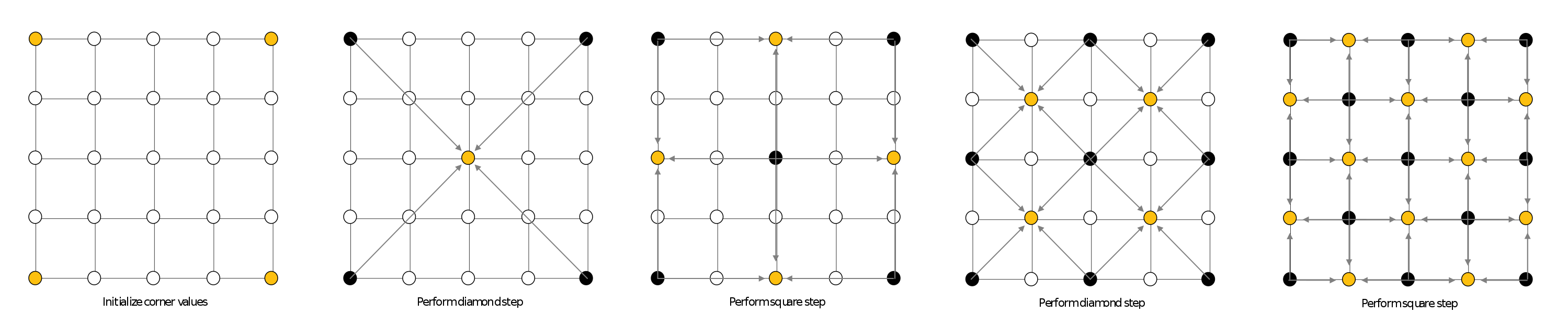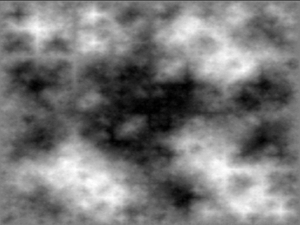# Fractal

naming: fractional dimension

## Definition:

Each different $$d_u results in a unique length, and as d_u approaches 0, the length measured approaches \infty The scale is related to length and this function describes the bumpiness of a fractal line. This is called Fractal Dimension. • Higher Fractal Dimension means more bumpiness • Lower Fractal Dimension means less bumpiness ### Shoreline/Mountain Topology Use fractal dimension to model a bumpy line, and computationally derive the line, rather than describing more details. ## Statistically Self-similar Recursive Tree: Tree := Stick + Tree + Tree Moreover, we need to take care of the angles, length and returning position. It becomes: - Stick - Turn - Tree -> this will expand to the same routine - Turn - Tree -> this will expand to the same routine - Turn - Backwards ### L-System The above process can be described by CFG:$$T:S\leftarrow T \rightarrow \rightarrow T \leftarrow \overline{S}$This use of CFG is called L-System. ### Improvement to L-System Tree • Randomness : This introduces Statistically similar fractal image. The sub-parts are statistically similar to the original image, but not exactly the same. To create a nice tree, it is important to examine each tree. There is a grammar to each type of tree, describing its patterns. However, sadly trees don’t grow by the fractal model. ## Incorporating Randomness Into Fractals Example Algorithm (Subdivide and Offset) repeat foreach segment offset midpoint for random distance hence create two segments • deeper repetition: bumpier More sophisticated examples: ### Height Map A raster vector of height • Can represent any terrain with no overlapping height (not bumpy topology, water, etc.) • Cannot represent extremely rocky terrain Diamond Square• Level 0: Four corners set to $$h = 0 • Level 1: Mid-point$$m_1$ set to $h_1 += random$
• Level 2: Point on Edges aligned with m_1\$, offset randomly
• Level 3: Mid-point of each sub-square, offset randomly

The result could look something like:The problem is the grid pattern is visible. There are ‘+’ in the graph, so rotating the graph will be noticed (not entirely natural)

Solution: Use

Applications:

• NOT good for terrain. Natural terrain has very few local minima. The minima will drain, and become global minima. This is not reflected by diamond square subdivision.

• Clouds. Clouds density is similar to the patterns generated from diamond square subdivision.

• Dirt. This could create “dirty” texture to human-created objects.

### Perlin Fractal

A perlin fractal is created by taking in a height map, downsize it, and fill itself with the small height map.